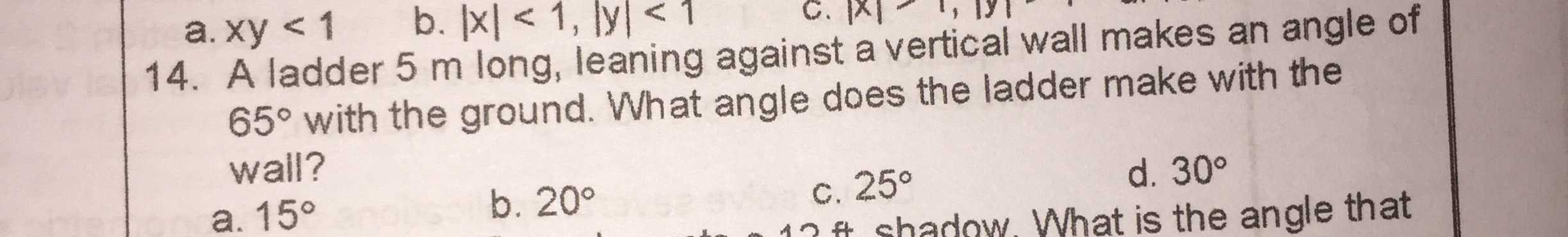### ¿Todavía tienes preguntas de matemáticas?

Pregunte a nuestros tutores expertos
Algebra
Pregunta14. A ladder $$5 m$$ long, leaning against a vertical wall makes an angle of $$65 ^ { \circ }$$ with the ground. What angle does the ladder make with the wall?

a. $$15 ^ { \circ }$$ b. $$20 ^ { \circ }$$ c. $$25 ^ { \circ }$$ d. $$30 ^ { \circ }$$

$$c$$